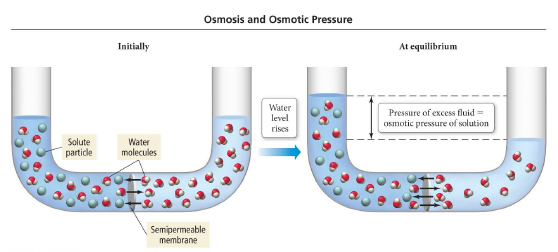# Problem: Which of the following solutions will have the largest osmotic pressure?a) 0.50 m C6H12O6b) 0.50 m NaClc) 0.50 m K2SO4d) 0.50 m FeCl3

###### FREE Expert Solution
90% (240 ratings)
###### FREE Expert Solution

We’re being asked to determine the solution with the highest osmotic pressure.

The osmotic pressure (Π) of a solution is related to the molarity of the solution:

$\overline{){\mathbf{\Pi }}{\mathbf{=}}{\mathbf{iMRT}}}$

where:

i = van’t Hoff factor

M = molarity of the solution (in M or mol/L)

R = gas constant (0.08206 atm • L/mol • K)

T = temperature

90% (240 ratings)###### Problem Details

Which of the following solutions will have the largest osmotic pressure?

a) 0.50 m C6H12O6

b) 0.50 m NaCl

c) 0.50 m K2SO4

d) 0.50 m FeCl3What scientific concept do you need to know in order to solve this problem?

Our tutors have indicated that to solve this problem you will need to apply the The Colligative Properties concept. You can view video lessons to learn The Colligative Properties. Or if you need more The Colligative Properties practice, you can also practice The Colligative Properties practice problems.

What is the difficulty of this problem?

Our tutors rated the difficulty ofWhich of the following solutions will have the largest osmot...as medium difficulty.

How long does this problem take to solve?

Our expert Chemistry tutor, Dasha took 3 minutes and 26 seconds to solve this problem. You can follow their steps in the video explanation above.

What professor is this problem relevant for?

Based on our data, we think this problem is relevant for Professor Bindell's class at UCF.